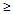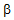# Electronic Devices - BJT Amplifiers

### Exercise :: BJT Amplifiers - Filling the Blanks

11.

In a voltage-divider bias configuration, the voltage-divider equation is used to determine the ________.

 A. ac level of Vb B. dc level of IB C. dc level of VB D. ac level of Ib

Explanation:

No answer description available for this question. Let us discuss.

12.

The ________ of the input signal is one of the first concerns in the sinusoidal ac analysis of transistor networks.

 A. period B. frequency C. magnitude D. None of the above

Explanation:

No answer description available for this question. Let us discuss.

13.

The ________ model(s) is (are) commonly used in the small-signal ac analysis of transistor networks.

 A. re B. hybrid equivalent C. re and hybrid equivalent D. None of the above

Explanation:

No answer description available for this question. Let us discuss.

14.

In a voltage-divider bias configuration, there can be a measurable difference in the results for ________ if the condition ro10RC is not satisfied.

 A. Zo B. Av C. Ai D. All of the above

Explanation:

No answer description available for this question. Let us discuss.

15.

The level of re is determined by ________.

 A. a B. IE C.D. IB